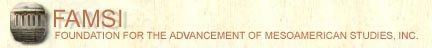John Montgomery
Dictionary of Maya Hieroglyphs

﻿

M

To hear the sounds and learn more, click on the text below the glyph.

Search the dictionary for words in English or Maya.
Please be sure java is enabled on your browser.

A | B' | CH | CH' | E | H | I | J | K | K' | L | M | N | O | P | S | T | T' | TZ | TZ' | U | W | X | Y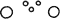ma (ma)
(T140)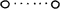ma (ma)
(T140)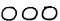ma (ma)
(T142)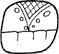ma (ma)
(T566)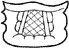ma (ma)
(T566)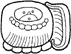ma-ka (mak/Mak)
(T502:314.25)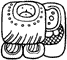ma-ka-ja (makaj)
(T502:25.25.181)ma-ka-xa (makax)
(T502:25.25.114)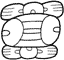ma-ko-ma (makom)
(T74:110:74)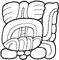ma-ta-wi-la (matawil)
(T74:565a:178.117)ma-xi (max)
(T502:142 736v)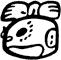ma-xi (max)
(T74:736v)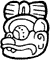ma-xi (max)
(T74:736v)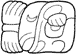MA-[IL]-aj (ma ilaj)
(T74.743)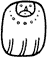ma/MA (ma)
(T502)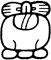ma/MA (ma)
(T74:501:142)ma/MA (ma)
(T74)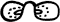ma/MA (ma)
(T74)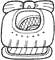MAK (Mak)
(T74:501v:25)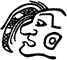MAM (mam)
(T1014v)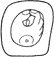MANIK' (Manik')
(T671)MAY(?) (may ?)
(Tnn)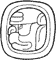MEN (Men)
(T1017v?)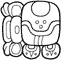MI-OL-la (mi ol)
(T173.506.178)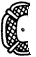mi/MI (mi)
(T173)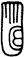mi/MI (mi)
(T217v)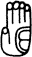mi/MI (mi)
(T217v)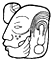mi/MI (mi)
(Tnn)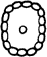mo (mo)
(T582)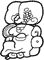MO' WITZ (Mo' Witz)
(T743v:1030p)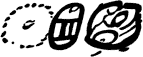mo-ko-chi (mokoch)
(T582.110:671)mo-o WITZ AJAW (Mo' Witz Ajaw)
(T582.279.168:529)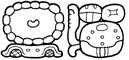mo-o wi-tzi AJAW (Mo' Witz Ajaw)
(T582.279 117.168:507)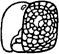mo/MO' (mo/mo')
(T743v)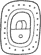MOL (Mol)
(T581)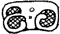MOL(?) (mol?)
(T5)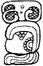MOL(?) TUN-ni (mol tun?)
(T5:528:116)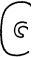mu (mu)
(T19)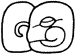mu (mu)
(T19.741)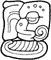mu-ka (muk)
(T19.741:25)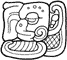mu-ka-ja (mukaj)
(T19.174:25.181)mu-ka-ja (mukaj)
(T19.25:1000a)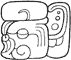mu-ka-ja (mukaj)
(T19:738c.181)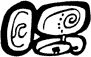mu-ku-yi (mukuy)
(T19.528:17)mu-ti (mut)
(T19:59)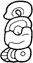mu-ti (mut)
(T19:741v:59)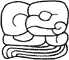mu-wa-ni (muwan)
(T19.738:130:116)MULUK (Muluk)
(T513)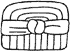MUTUL (mutul)
(T569)MUTUL (mutul)
(T778)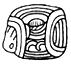MUTUL (mutul)
(T778v)MUTUL AJAW-TAK (mutul ajaw-tak)
(T569.168:559)MUWAN-na (muwan)
(T744:23)MUWAN-ni (Muwan)
(T744:116)MUYAL (muyal)
(T632v)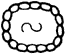MUYAL (muyal)
(T632v)

Index | Next Page
Return to top of page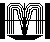Order John Montgomery’s Book